# ISEE Middle Level Math : How to find the part from the whole

## Example Questions

### Example Question #11 : How To Find The Part From The Whole

Ninety-six people must form eight equal groups. How many people will be in each group?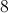Explanation:

Divide: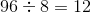Answer: There will be 12 people in each group.

### Example Question #181 : Whole And Part

Three friends are in the desert and must share a 15 ounce bottle of water. How many ounces of water will each friend drink, assuming they split the bottle evenly?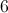Explanation:

Divide: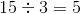Answer: Each friend will drink 5 ounces of water.

### Example Question #11 : How To Find The Part From The Whole

Pamela takes her children to the fair. How many $3.00 tickets can she buy if she only has$21.00?Explanation:

Divide: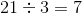### Example Question #942 : Isee Middle Level (Grades 7 8) Mathematics Achievement

In March, there were 10 sunny days, 10 cloudy days, and 11 rainy days. How many days did it rain in March?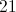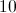Explanation:

Identify the number of rainy days in March:days

### Example Question #12 : How To Find The Part From The Whole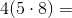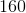Explanation:

Solve inside the parentheses: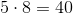Then, solve: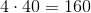### Example Question #54 : Numbers And Operations

Sal is going to the fair with his family. He has $18.00 to buy tickets. How many tickets can he buy if each one costs$2.25?Explanation:

Divide the total amount of money he has by the cost of one ticket: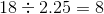### Example Question #24 : How To Find The Part From The Whole

Julie gets paid $304 a week for dogsitting. If she wants to buy a new washing machine that costs$1400, how many weeks does Julie need to work to save up the required amount? Round to the nearest whole week.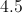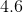Explanation:

Divide the cost of the washing machine by the amount of money she makes each week: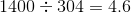Answer: Julie needs to save for 5 weeks.

### Example Question #55 : Numbers And Operations

In order to travel from New York to Florida with his family, Eric needs to purchase 3 tickets, costing $52.00,$64.79 and $44.50. If he has$213.00 in his wallet, how much money will Eric have left over after buying the tickets?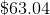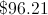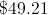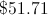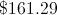Explanation:

First add up the prices of the three tickets: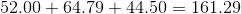Then subtract that amount from $213: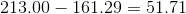Therefore Eric will have$51.71 left over.

### Example Question #11 : How To Find The Part From The Whole

Natasha is going Christmas shopping for the twelve people on her list. On average, how much money can she spend per gift if she has a $225.00 budget? Possible Answers: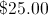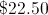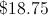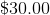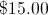Correct answer:Explanation: Divide$225 by 12: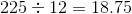### Example Question #61 : Numbers And Operations

Which of the following is a factor of 72?None of theseExplanation:

Factors can be multiplied to get a certain number; when a certain number is divided by a factor, the result is a whole number.

When 18 and 4 are multiplied, the result is 72; thus, 18 and 4 are both factors of 72.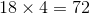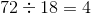When 72 is divided by the other answer choices, a whole number does NOT result.

### All ISEE Middle Level Math Resources